# GRE Subject Test: Math : Tests for Convergence

## Example Questions

### Example Question #1 : Ratio Test

Which of these series cannot be tested for convergence/divergence properly using the ratio test? (Which of these series fails the ratio test?)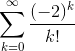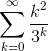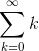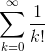Explanation:

The ratio test fails when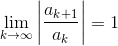. Otherwise the series converges absolutely if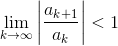, and diverges if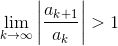.

Testing the series, we have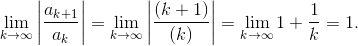Hence the ratio test fails here. (It is likely obvious to the reader that this series diverges already. However, we must remember that all intuition in mathematics requires rigorous justification. We are attempting that here.)

### Example Question #2 : Ratio Test

Assuming that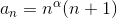,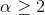. Using the ratio test, what can we say about the series: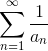We cannot conclude when we use the ratio test.

It is convergent.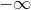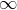We cannot conclude when we use the ratio test.

Explanation:

As required by this question we will have to use the ratio test.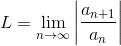if L<1 the series converges absolutely, L>1 the series diverges, and if L=1 the series could either converge or diverge.

To do so, we will need to compute :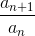. In our case: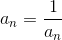Therefore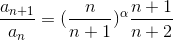.

We know that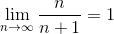This means that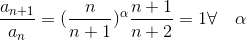Since L=1 by the ratio test, we can't conclude about the convergence of the series.

### Example Question #1 : Ratio Test

We consider the series :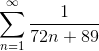, use the ratio test to determine the type of convergence of the series.

The series is fast convergent.

We cannot conclude about the nature of the series.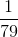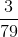It is clearly divergent.

We cannot conclude about the nature of the series.

Explanation:

To be able to use to conclude using the ratio test, we will need to first compute the ratio then useif L<1 the series converges absolutely, L>1 the series diverges, and if L=1 the series could either converge or diverge. Computing the ratio we get,.

We have then: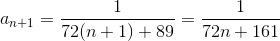Therefore have :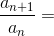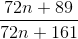It is clear that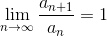.

By the ratio test , we can't conclude about the nature of the series.

### Example Question #183 : Gre Subject Test: Math

Consider the following series :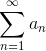where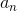is given by: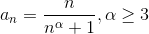. Using the ratio test, find the nature of the series.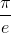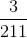We can't conclude when using the ratio test.The series is convergent.

We can't conclude when using the ratio test.

Explanation:

Letbe the general term of the series. We will use the ratio test to check the convergence of the series.if L<1 the series converges absolutely, L>1 the series diverges, and if L=1 the series could either converge or diverge.

We need to evaluate,we have: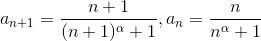.

Therefore: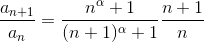. We know that,and therefore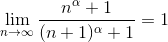This means that :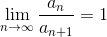.

By the ratio test we can't conclude about the nature of the series. We will have to use another test.

### All GRE Subject Test: Math Resources